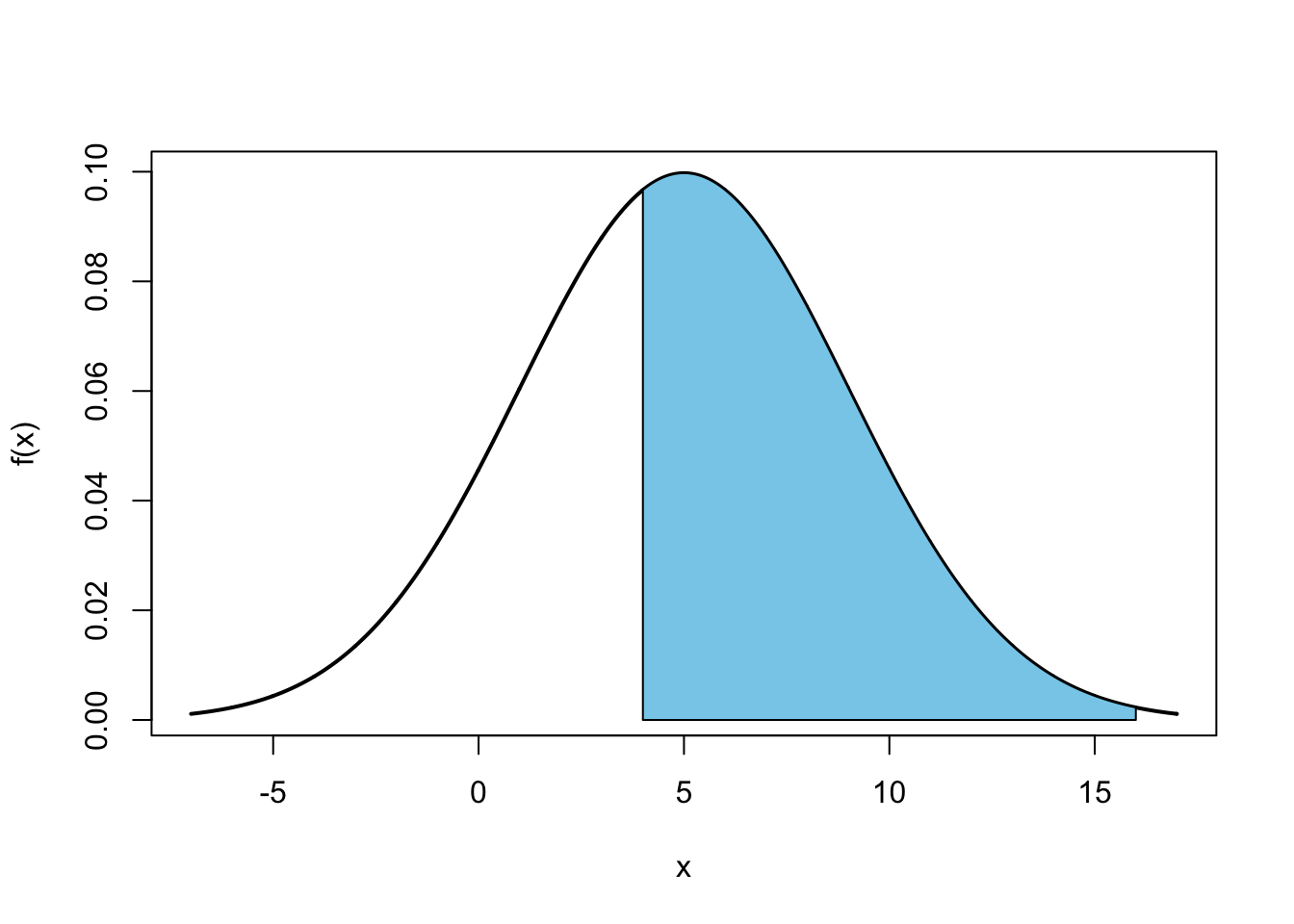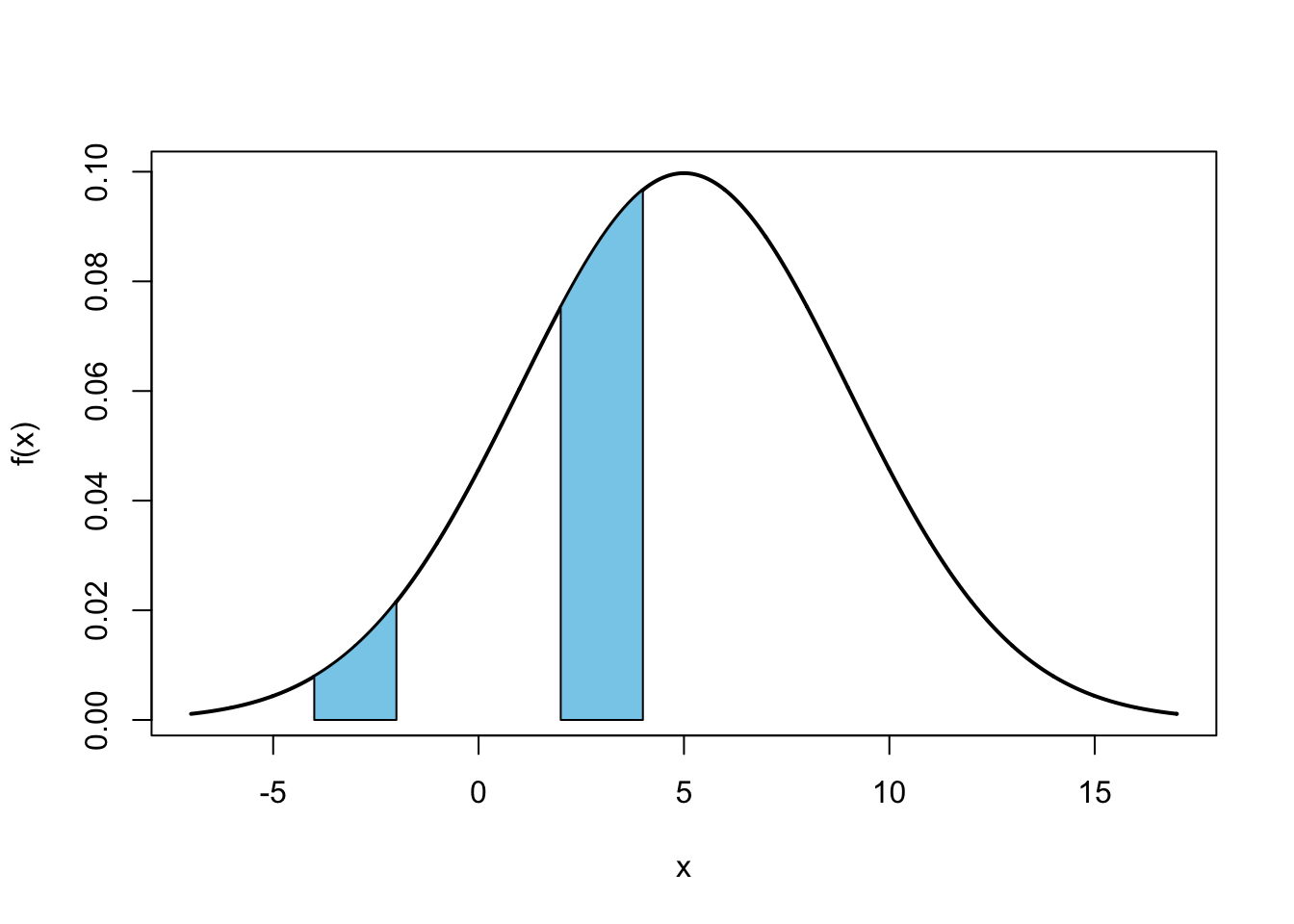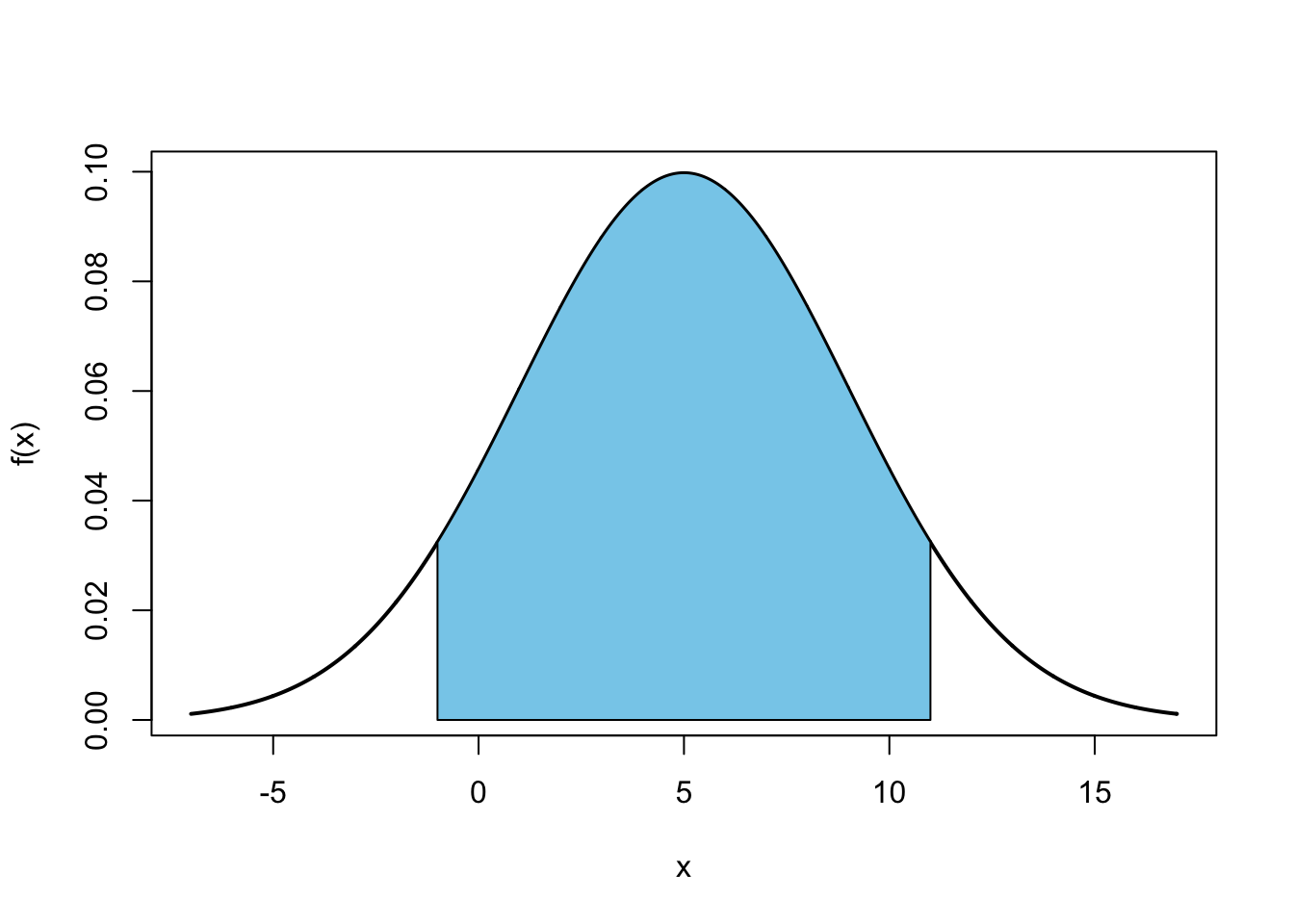## Exercise 1

Consider a random variable $$X$$ with the moment generating function

$M_X(t) = e^{5t + 8t^2} = \exp(5t + 8t^2)$

(a) Calculate $$P(4 < X < 16)$$.

Solution:

Since the moment generating function for a normal random variable $$X$$, with mean $$\mu$$ and variance $$\sigma^2$$ is given by

$M_X(t) = e^{\mu t + \sigma^2 t^2 / 2}$

we know that the moment generating function given is that of a normal random variable with

$\mu = 5$

and

$\frac{\sigma^2}{2} = 8$

Thus we have

$\text{E}[X] = 5$

and

$\text{Var}[X] = 16.$

Then

\begin{aligned} P(4 < X < 16) &= P\left(\frac{4 - 5}{4} < Z < \frac{16 - 5}{4}\right) = P(-0.25 < Z < 2.75)\\ &= P(Z < 2.75) - P(Z < -0.25) = 0.9970 - 0.4013 = \boxed{0.5957} \end{aligned}c(pnorm(-0.25), pnorm(2.75))
##  0.4012937 0.9970202
(pnorm(2.75) - pnorm(-0.25))
##  0.5957266
diff(pnorm(c(4, 16), mean = 5, sd = 4))
##  0.5957266

(b) Calculate $$P(4 < X^2 < 16)$$.

Solution:

\begin{aligned} P(4 < X^2 < 16) &= P(-4 < X < -2) + P(2 < X < 4)\\ &= P(-2.25 < Z < -1.75) + P(-0.75 < Z < -0.25)\\ &= (0.0401 - 0.0122) + (0.4013 - 0.2266) = \boxed{0.2026} \end{aligned}c(pnorm(-2.25), pnorm(-1.75), pnorm(-0.75), pnorm(-0.25))
##  0.01222447 0.04005916 0.22662735 0.40129367
(pnorm(-1.75) - pnorm(-2.25)) + (pnorm(-0.25) - pnorm(-0.75))
##  0.202501
diff(pnorm(c(-4, -2), mean = 5, sd = 4)) +
diff(pnorm(c(2, 4), mean = 5, sd = 4))
##  0.202501

## Exercise 2

Consider a random variable $$X$$ with $$\text{E}[X] = 5$$ and $$\text{Var}[X] = 16$$.

(a) Calculate $$P(|x - 5| < 6)$$ if $$X$$ follows a normal distribution.

Solution:

\begin{aligned} P(|X - 5| \leq 6) &= P[|X - 5| \leq (1.5) \cdot (4)] \\ &= P(-1.5 < Z < 1.5)\\ &= P(Z < -1.5) - P(Z < 1.5)\\ &= 0.9332 - 0.0668\\ &= \boxed{\bf{0.8664}} \end{aligned}diff(pnorm(c(-1, 11), mean = 5, sd = 4))
##  0.8663856

(b) Use Chebyshev’s inequality to provide a lower bound for $$P(|x - 5| < 6)$$. (No longer assume $$X$$ is normal.)

Solution:

\begin{aligned} P(|X - 5| \leq 6) &= P(|X - 5| \leq (1.5) \cdot(4)) \\[1ex] &\geq 1 - \frac{1}{(1.5)^2}\\[1ex] &= \boxed{\bf{0.5556}} \end{aligned}

## Exercise 3

In the original Pokémon Red and Blue, there were 151 Pokémon, but only 150 of these Pokémon could actually be caught or obtained in the games. If you wanted to “catch ’em all”, the 151st Pokémon, Mew, could only be obtained through special giveaway events at local video game retailers. (For example FuncoLand, which was later purchased by GameStop.)

Suppose that these giveaway events at your local FuncoLand occur according to a Poisson process with an average of one event per two months.

For this exercise, assume that all 12 months of the year have the same number of days. Additionally, assume that the four seasons all last exactly three months.

• Winter: December, January, February
• Spring: March, April, May
• Summer: June, July, August
• Fall: September, October, November

Also, suppose that it is the beginning of a new year, that is January 1, 1999.

(a) What is the probability that the first event occurs before Spring?

Solution:

Define:

• $$T_k$$ as the waiting time (in months) until the $$k$$th event
• $$X_t$$ as the number of events in time (months) $$t$$

Here, $$T_1$$ follows an exponential distribution with $$\lambda = 0.5$$.

$P(T_1 < 2) = 1 - e ^ {-(0.5)(2)} = \boxed{0.6321}$